# Area of Triangles Problems with Solutions

A set of problems on how to calculate the area of triangles using different formulas are presented along with detailed solutions.

## Formulas for Area of Triangles

We first recall some of the most widely used formulas used to calculate the area of a triangle.

### Formula 1 - Base and Height of a triangle are known

When a base and the corresponding height are known, the area A of the triangle may be calculated as follows: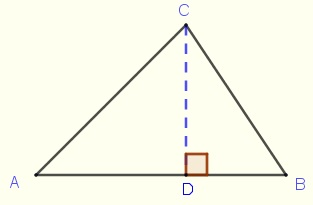Area = (1 / 2) � base � height = (1 / 2) � AB � CD

### Formula 2 - Two sides of a triangle and the angle between them are known

When two sides and the angle between them are known, the area A of the triangle may be calculated as follows:Area = (1 / 2) � CA � CB � sin (C)

### Formula 3 - Three sides of a triangle are known

When the lengths a = CB, b = CA and c = AB of all three sides of a triangle are known, the area A of a triangle may be calculated using Heron's formula:
Area = √[ s � (s - a) � (s - b) � (s - c) ]

where s in the above formula is given by s = (1 / 2)(a + b + c)

### Formula 4 - The three vertices of the triangle are known by their coordinates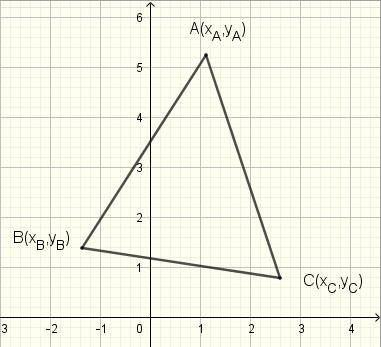If A(xA , yA), B(xB , yB) and C(xC , yC) are the three vertices of a triangle given by their coordinates, then the formula for the area of the triangle defined by the three vertices A, B and C is given by:where det is the
determinant of the three by three matrix.

## Problems on Areas with Solutions

Problem 1
Find the area of each of the triangles shown below.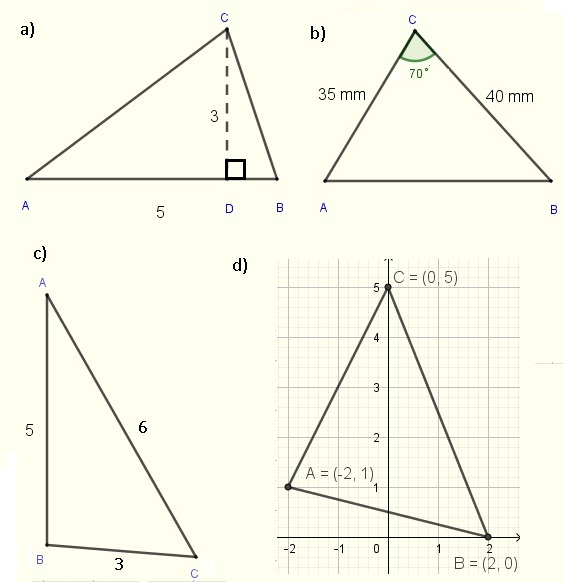Problem 2
Find the area of triangle CDB in the figure below.Problem 3
Find the area of the triangle below.Problem 4
Find the area of an equilateral triangle wide length equal to 6 cm.

Problem 5
In the figure below, ABCD is a rectangle of length of length 60 and width 30. The length of of BF and GC are respectively equal to 10 and 20. Find the area of the shaded region.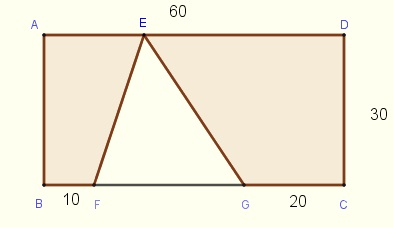Problem 6
The quadrilateral FGHE is inscribed in the rectangle ABCD of length 100 and width 50 such that E is the midpoint of segment AB and G is the midpoint of segment DC. Find the area of the quadrilateral FEHG.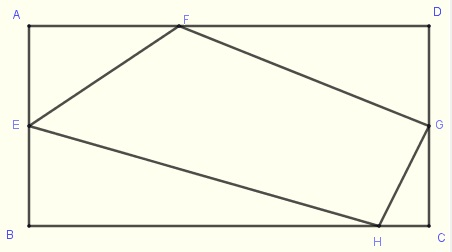Problem 7
Find the area of the triangle whose vertices are the points of intersection of the lines with equations y = x , y = - x + 4 and y = 2 x + 2.

Problem 8
Find the area of the shape below.## Solutions to the Above Problems

Solution to Problem 1
a) The base and height of the triangle in part a) are known, hence the use of formula 1.
Area = (1 / 2) � 5 � 3 = 7.5 unit 2
b) Two sides and the angle between them of the triangle in part b) are known, hence the use of formula 2.
Area = (1 / 2) � 35 � 40 � sin(70�) = 657.8 mm 2
c) All three sides of the triangle in part c) are known, hence the use of formula 3.
s = (1/2)(5 + 3 + 6) = 7
Area = √( 7(7 - 5)(7 - 3)(7 - 6) ) = 7.5 unit 2
c) The vertices of the triangle in part c) are defined by their coordinates and we therefore use formula 4 to find of this triangle.
We use the coordinates of A(xA , yA), B(xB , yB) and C(xC , yC) to write matrix M as follows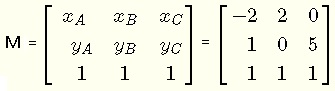We next find the determinant of matrix M
Det (M) = 18
The are of triangle in part d) = ( 1/2) |Det (M)| = 9 unit 2

Solution to Problem 2
Triangle CDB has a base CD of length 20. Its height is the side AB of the triangle because it start from the vertex B opposite the base CD is perpendicular to AC and therefore to the base DC. Hence the use of formula 1 to find the area of triangle.
Area = (1/2) � 20 � 80 = 40 unit 2

Solution to Problem 3
We are given two sides and the angle opposite one of the sides. One way to find the area is to find angle B and use formula 2.
Use the sine law to write
sin(A) / BC = sin(C) / AB
which gives
sin(A) = 6 sin(55�) / 5
A = sin-1(6 sin(55�) / 5) = 79.4 �
We now determine angle B using that fact that the sum of all angles in a triangle to be 180 �.
B = 180 - 55 - 79.4 = 45.6 �
Use formula 2 to find the area
Area = (1 / 2) � 5 � 6 � sin(45.6 �) = 10.7 unit 2

Solution to Problem 4
Let ABC be an equilateral triangle of side 6. Because the triangle is equilateral, the height from vertex A to the base BC splits the base BC into two segments of equal length of 3 unit as shown in the figure below.The length of the height AH is calculated using the Pythagorean theorem as follows:
AH 2 + 3 2 = 6 2
AH = √(27) = 3 √3
Use formula 1 to find the area of triangle ABC
Area of triangle AHC = (1 / 2) � AH � BC = (1 / 2) � 3 √3 � 6 = 15.6 unit 2

Solution to Problem 5
One way to find the area of the shaded region is to subtract the area of the triangle EFG from the area of the rectangle.
The length of the base FG of triangle EFG is obtained by subtracting the lengths of BF and CG from the length of the rectangle.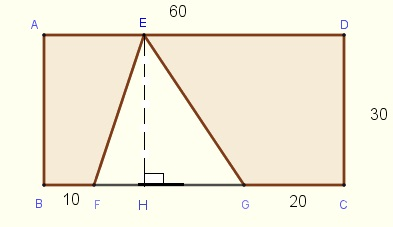FG = 60 - 10 - 20 = 30
The length of the height EH from vertex E of triangle EFG is equal to the width of the rectangle.
Area of triangle EFG = (1 / 2) � EH � FG = (1 / 2) � 30 � 30 = 450 unit 2
The area of the shaded region is obtained by subtracting the area of the triangle EFG from the area of the rectangle as follows
Area of the shaded region = 60 � 30 - 450 = 1350 unit 2

Solution to Problem 6
The area of the quadrilateral FGHE may be calculated by adding the ares of triangles FEG and EHG. These two triangles have a common base EG.
Since E and G are the midpoints of segments AB and DC respectively, we conclude that:
1) the length of the base EG is equal to the length of the rectangle which is equal to 100.
2) the length of the height FF' of triangle FEG and the length of the height HH' of triangle EHG are both equal to half the width of the rectangle which is 25.Area of triangles FEG = (1 / 2) base � height = (1 / 2) EG � FF' = (1 / 2) 100 � 25 = 1250
Area of triangles EHG = Area of triangles FEG = 1250
Area of the quadrilateral FGHE = 1250 + 1250 = 2500 unit 2

Solution to Problem 7
We graph the lines with equations y = x , y = - x + 4 and y = 2 x + 2 on a system of axis as shown below.We now have to find the vertices A, B and C as the intersection of the pairs of lines
1) Find A as the intersection of y = 2x + 2 and y = - x + 4
Solve the system y = 2x + 2 and y = - x + 4 by substitution
2x + 2 = - x + 4
gives the coordinates of A as: x = 2 / 3 and y = 5 / 2

2) Find B as the intersection of y = 2x + 2 and y = x Solve the system y = 2x + 2 and y = x by substitution 2x + 2 = x gives the coordinates of B as: x = - 2 and y = - 2

3) Find C as the intersection of y = x and y = - x + 4
Solve the system y = x and y = - x + 4 by substitution
x = - x + 4
gives the coordinates of C as: x = 2 and y = 2
Since we have the coordinates of the three vertices of the triangle, we may use formula 4 as follows
Area of triangle ABC =Solution to Problem 8
The shape whose are is to be calculated is made up of two triangles ADC and ACB with a common side AC. The area of triangle ACB can be calculated using formula 2 (we know two sides and the angle between them).
For triangle ADC ,we know two sides. We can find side AC using the cosine law. in triangle ACB as follows
AC 2 = AB 2 + CB 2 - 2 (AB)(AC) cos (B)
Substitute by the numerical values and calculate AC
AC = √( 400 2 + 800 2 - 2 (400)(800) cos (45�) ) = 589.5
We may use Heron's formula since we know the three sides of triangle ADC
Let s = (1/2)(AD + DC + CA) = (1/2)(245 + 432 + 589.5) = 633.25
Area of triangle ADC = √( s � (s - AD) � (s - DC) � (s - CA) )
= √( 633.25 � (633.25 - 245) � (633.25 - 432) � (633.25 - 589.5) ) = 46526 ft 2
Area of triangle ACB = (1 / 2) BA � BC � sin (B) = (1 / 2) 400 � 800 � sin (45�) = 113137 ft 2
Total area of the given shape is obtained by adding the areas of triangles ADC and ACB
Area = 46526 + 113137 = 158663 ft 2